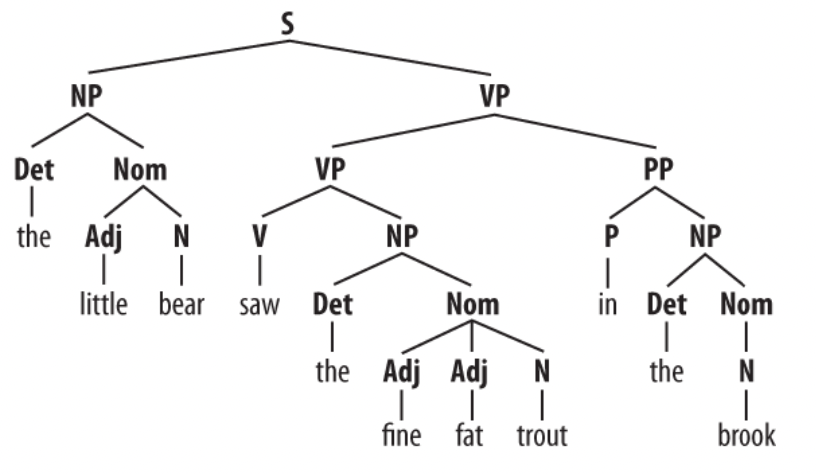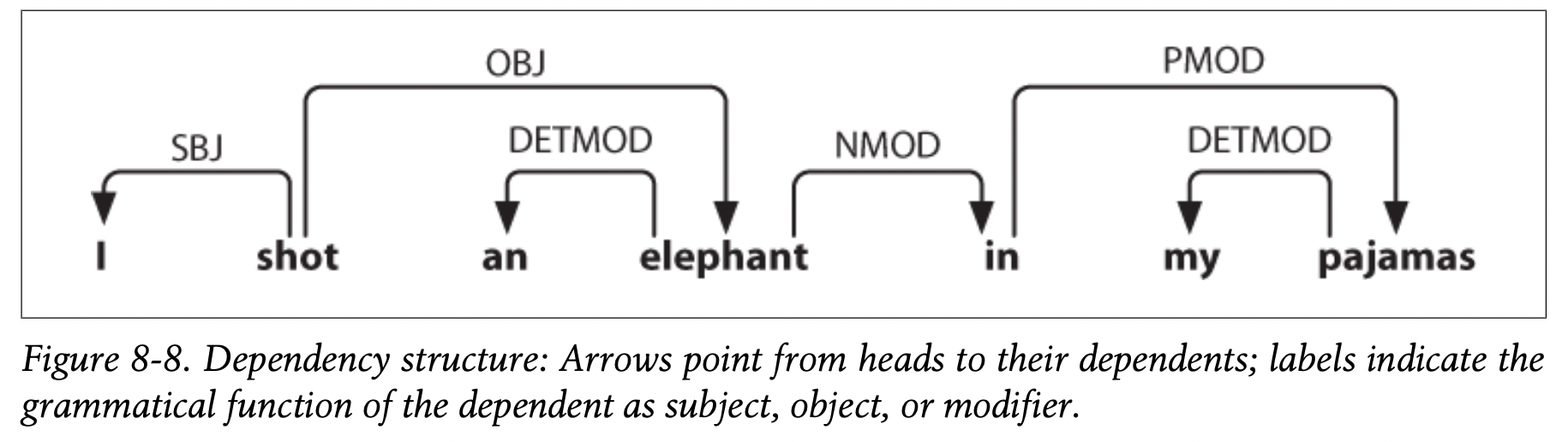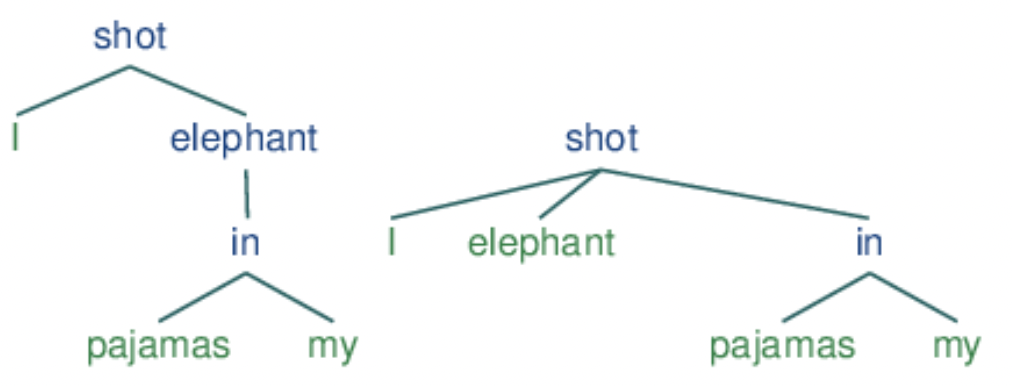nlp

Some notes on natural language processing, focused on modern improvements based on deep learning.

# benchmarks

Nice repo keeping track of progress here.

• tokenization - convert raw text into tokens that can be fed to a model
• token/word embeddings - convert tokens to semantic vectors
• pos tagging - give each word its part-speech
• named entity recognition - classify tokens based on some known annotations (e.g. person, organization)
• nested entity recognition - more complex than individual tokens (e.g. “Mary = “Jacob’s brother”)
• parse tree extraction - extract out tree of how words operate in a grammar
• sentiment classification
• text summarization
• language modeling (i.e. text generation)
• (machine) translation
• coreference resolution - correspond simple entities (e.g. “she = Mary”, different names for a protein)
• natural language inference - determining whether a “hypothesis” is true (entailment), false (contradiction), or undetermined (neutral) given a “premise”
• topic modeling - unsupervised learning over documents

## datasets

open-ended

very popular

• SuperGLUE Benchmark (2019)
• 8 NLU tasks (keeps two hardest from GLUE, marked with ***) extending to coreference resolution and question-answering
• BoolQ (Boolean Questions) - answer a yes/no question about a text passage.
• CB (CommitmentBank) - given a text and a clause, predict how much the text commits to the clause
• COPA (Choice of Plausible Alternatives) - given a premise sentence and two possible choices, the system must determine either the cause or effect of the premise from two possible choices
• MultiRC (Multi-sentence Reading Comprehension) - given a context paragraph, a question about that paragraph, and a list of possible answers, the system must predict which answers are true and which are false
• ReCoRD (Reading Comprehension with Commonsense Reasoning Dataset) - multiple-choice QA task (news article + Cloze-style multiple-choice question)
• $^\dagger$RTE (Recognizing Textual Entailment) - determine if a sentence entails a given hypothesis or not.
• WiC (Word-in-Context) - word sense disambiguation task cast as binary classification of sentence pairs. Given two text snippets and a polysemous word that appears in both sentences, the task is to determine whether the word is used with the same sense in both sentences
• $^\dagger$WSC (Winograd Schema Challenge) - coreference resolution task in which examples consist of a sentence with a pronoun and a list of noun phrases from the sentence. The system must determine the correct referent of the pronoun from among the provided choices.
• GLUE Benchmark (2019) = General language understanding evaluation
• 9 NLU tasks including question answering, sentiment analysis, text similarity and textual entailment
• CoLA (Corpus of Linguistic Acceptability) - determine if sentence is grammatically correct
• SST-2 (Stanford Sentiment Treebank) - determine if the sentence has a positive or negative sentiment
• MRPC (Microsoft Research Paraphrase Corpus) - determine if two sentences are paraphrases from one another.
• QQP (Quora Question Pairs)- determine if two questions are semantically equivalent or not.
• STS-B (Semantic Textual Similarity Benchmark) - determine the similarity of two sentences with a score from one to five.
• MNLI (Multi-Genre Natural Language Inference) - determine if a sentence entails, contradicts, or is unrelated to another sentence
• QNLI (Question-answering Natural Language Inference) - determine if the answer to a question is contained in a second sentence or not
• RTE (Recognizing Textual Entailment) - determine if a sentence entails a given hypothesis or not
• WNLI (Winograd Natural Language Inference) - determine if a sentence with an anonymous pronoun and a sentence with this pronoun replaced are entailed or not
• more NLI ( natural language inference)
• ANLI: Adversarial NLI (nie et al. 2019) - harder examples found by model failures
• SNLI Benchmark (bowman et al. 2015) = Stanford Natural Languge Inference - entailment dataset
• FEVER: Fact Extraction and VERification (Thorne et al., 2018)
• SciTail (khot et al. 2018) - textual entailment derived from science-question answering
• QA
• SQuAD (Rajpurkar…liang, 2016) - Stanford Question Answering Dataset (SQuAD) - 100k questions from 23k passages in 500 wikipedia articles

common data sources

• WSJ
• then Wikipedia

## eval metrics

• perplexity (PP) - inverse probability of the test set, normalized by the number of words (want to minimize it)

• $PP(W_{test}) = P(w_1, …, w_N)^{-1/N}$
• can think of this as the weighted average branching factor of a language
• should only be compared across models w/ same vocab
• BLEU
• bert-score: https://github.com/Tiiiger/bert_score

## tokenization

Tokenizers - Hugging Face Course

• word-based
• punctuation splitting
• unknown token [UNK] for anything not seen - to reduce the amount of this, can get character-based tokens
• vocab tends to be too big
• subword-based - break apart meaningful subparts of words, most popular (2022)
• many more (e.g. byte-level BPE, used in GPT-2)
• character-based - very little prior, generally sequences are too long
• vocabulary
• sometimes closed, otherwise have unkown words, which we assign its own symbol
• can fix training vocab, or just choose the top words and have the rest be unkown
• preprocessing
• stemming - maps words to a common root, usually by just chopping off the ending (e.g. “dogs” -> “dog”)
• most common way to do this is Porter’s algorithm, which has 5 phrases of rule-based word reductions
• lemmatization - smarter version that takes into account vocab / morphological analysis (e.g. “am, are, is” -> “be”)

## token / word embeddings

embeddings - vectors for representing words

• ex. tf-idf - defined as counts of nearby words (big + sparse)
• TF * IDF = [ (Number of times term t appears in a document) / (Total number of terms in the document) ] * log(Total number of documents / Number of documents with term t in it).
• pointwise mutual info - instead of counts, consider whether 2 words co-occur more than we would have expected by chance
• ex. word2vec - short, dense vectors
• intuition: train classifier on binary prediction: is word $w$ likely to show up near this word? (algorithm also called skip-gram)
• the weights are the embeddings
• word2vec paper I: initial word2vec (mikolov et al. 2013) - simplifies neural language models for efficient training of word embeddings
• maximizing the probabilities of words being predicted by their context words (with a DNN)
• continuous bag-of-words (CBOW) - predict current word from window (order doesn’t matter)
• skipgram - use current word to predict surrounding window – nearby context words weighted more heavily
• word2vec paper II: Distributed Representations of Words and Phrases and their Compositionality (mikolov et al. 2013) - word2vec improvements
• identify key ngrams and give them their own vecs
• ex. GloVe (pennington, socher, & manning, 2014), which is based on ratios of word co-occurrence probs
• ex. ELMO (peters…zettlemoyer, 2022) - use LSTM for word embeddings

## language modeling

language models - assign probabilities to sequences of words

• ex. n-gram model - assigns probs to short sequences of words, known as n-grams
• for full sentence, use markov assumption
• multi-token decoding for classification - regular beam search will favor shorter results over longer ones on average since a negative log-probability is added at each step, yielding lower (more negative) scores for longer sentences

## topic modeling

topic models (e.g. LDA) - apply unsupervised learning on large sets of text to learn sets of associated words

• LDA = latent dirichlet allocation

## grammar / parse-tree exraction

notes/figures from nltk book ch 8/9

• language - set of all grammatical sentences
• grammar - formal notation that can be used for “generating” the members of this set
• phrases
• noun phrases
• structures
• constituent structure - words combine with other words to form units which are substitutable
• e.g. the fact that we can substitute He for The little bear indicates that the latter sequence is a unit
• coordinate structure: if $v_{1}$ and $v_{2}$ are both phrases of grammatical category $X$, then $v_{1}$ and $v_{2}$ is also a phrase of category $X$
• context-free grammar - set of grammar rules that define a gramm
• e.g. $S \to NP\; VP$
• rules can be probabilities (so we search for the most probable parse tree, rather than returning all)
• phrase tree
•• $\begin{array}{lll}\text { Symbol } & \text { Meaning } & \text { Example } \ \text { S } & \text { sentence } & \text { the man walked } \ \text { NP } & \text { noun phrase } & \text { a dog } \ \text { VP } & \text { verb phrase } & \text { saw a park } \ \text { PP } & \text { prepositional phrase } & \text { with a telescope } \ \text { Det } & \text { determiner } & \text { the } \ \text { N }& \text { noun } & \text { dog } \ \text { V } & \text { verb } & \text { walked } \ \text{ P} & \text { preposition } & \text { in }\end{array}$
• note: chunking yields a similar partition of a sequence rather than a tree
• algorithms
• top-down parsing (e.g. recursive descent parsing) - starts from $S$ , and keep expanding the grammar rules until finding a match
• RecursiveDescentParser is unable to handle left-recursive productions of the form X -> X Y
• bottom-up parsing - can be faster; find sequences that correspond to the righthand side of a grammar rule and replace them with the left
• ex. shift-reduce parser
• backtracking ensures we find a parse if one exists (and can find multiple if we choose)
• dependency grammar focuses on how words relate to other words
• relation between head (usually tensed verb) and its dependents (rest of the words)
•• this can also be displayed as a tree
•• a dependency graph is projective if, when all the words are written in order, the edges can be drawn above the words without crossing
• equivalent to saying that a word and all its descendants (dependents and dependents of its dependents, etc.) form a contiguous sequence of words within the sentence
• above graph is projective
• valencies - sometimes certain things in a class are allowed buothers are not
• e.g. “Buster was frightened” ✅ but “Buster saw frightened” :x:
• need subcategories of things (e.g. intransitive verb vs transitive verb vs dative verb) to know what symbols are allowed in rules
• verbs specifically have complements associated with them – unlike modifiers (like the word “really”), complemetes are not optional and usually not selected in the same way by the head
• feature-based grammars - add things to word representations (e.g. plurality) and use these in the grammar rules